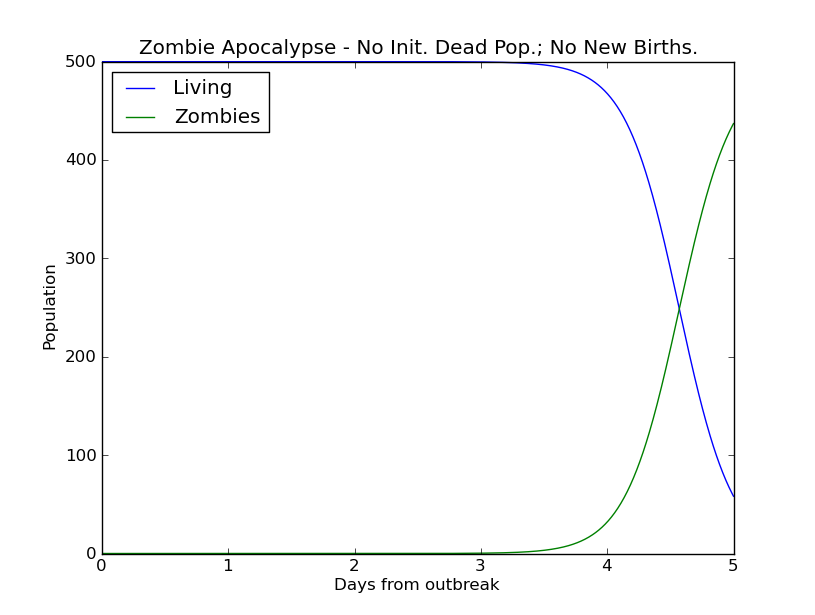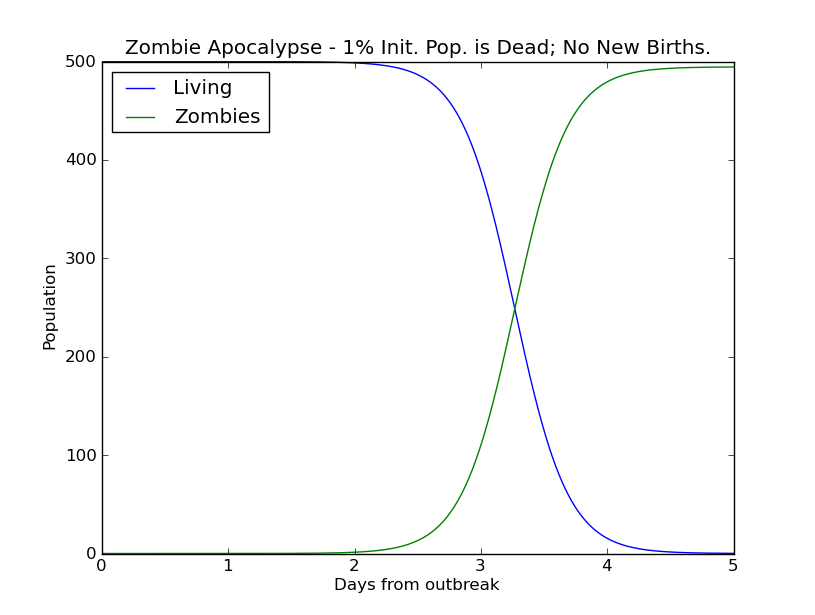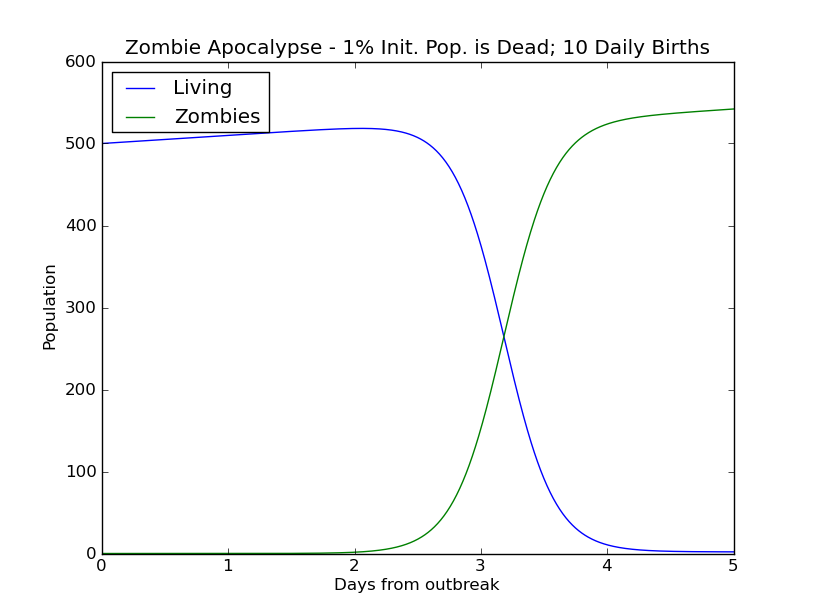This is an archival dump of old wiki content --- see scipy.org for current material.

## Modeling a Zombie Apocalypse

This example demonstrates how to solve a system of first order ODEs using SciPy. Note that a Nth order equation can also be solved using SciPy by transforming it into a system of first order equations. In a this lighthearted example, a system of ODEs can be used to model a "zombie invasion", using the equations specified in Munz et al. 2009.

The system is given as:

```dS/dt = P - B*S*Z - d*S
dZ/dt = B*S*Z + G*R - A*S*Z
dR/dt = d*S + A*S*Z - G*R```

with the following notations:

• S: the number of susceptible victims
• Z: the number of zombies
• R: the number of people "killed"
• P: the population birth rate
• d: the chance of a natural death
• B: the chance the "zombie disease" is transmitted (an alive person becomes a zombie)
• G: the chance a dead person is resurrected into a zombie
• A: the chance a zombie is totally destroyed

This involves solving a system of first order ODEs given by: dy/dt = f(y, t)

Where y = [S, Z, R].

The code used to solve this system is below:

```   1 # zombie apocalypse modeling
2 import numpy as np
3 import matplotlib.pyplot as plt
4 from scipy.integrate import odeint
5 plt.ion()
6
7 P = 0       # birth rate
8 d = 0.0001  # natural death percent (per day)
9 B = 0.0095  # transmission percent  (per day)
10 G = 0.0001  # resurect percent (per day)
11 A = 0.0001  # destroy percent  (per day)
12
13 # solve the system dy/dt = f(y, t)
14 def f(y, t):
15         Si = y
16         Zi = y
17         Ri = y
18         # the model equations (see Munz et al. 2009)
19         f0 = P - B*Si*Zi - d*Si
20         f1 = B*Si*Zi + G*Ri - A*Si*Zi
21         f2 = d*Si + A*Si*Zi - G*Ri
22         return [f0, f1, f2]
23
24 # initial conditions
25 S0 = 500.               # initial population
26 Z0 = 0                  # initial zombie population
27 R0 = 0                  # initial death population
28 y0 = [S0, Z0, R0]       # initial condition vector
29 t  = np.linspace(0, 5., 1000)   # time grid
30
31 # solve the DEs
32 soln = odeint(f, y0, t)
33 S = soln[:, 0]
34 Z = soln[:, 1]
35 R = soln[:, 2]
36
37 # plot results
38 plt.figure()
39 plt.plot(t, S, label='Living')
40 plt.plot(t, Z, label='Zombies')
41 plt.xlabel('Days from outbreak')
42 plt.ylabel('Population')
43 plt.title('Zombie Apocalypse - No Init. Dead Pop.; No New Births.')
44 plt.legend(loc=0)
45
46 # change the initial conditions
47 R0 = 0.01*S0   # 1% of initial pop is dead
48 y0 = [S0, Z0, R0]
49
50 # solve the DEs
51 soln = odeint(f, y0, t)
52 S = soln[:, 0]
53 Z = soln[:, 1]
54 R = soln[:, 2]
55
56 plt.figure()
57 plt.plot(t, S, label='Living')
58 plt.plot(t, Z, label='Zombies')
59 plt.xlabel('Days from outbreak')
60 plt.ylabel('Population')
61 plt.title('Zombie Apocalypse - 1% Init. Pop. is Dead; No New Births.')
62 plt.legend(loc=0)
63
64 # change the initial conditions
65 R0 = 0.01*S0   # 1% of initial pop is dead
66 P  = 10        # 10 new births daily
67 y0 = [S0, Z0, R0]
68
69 # solve the DEs
70 soln = odeint(f, y0, t)
71 S = soln[:, 0]
72 Z = soln[:, 1]
73 R = soln[:, 2]
74
75 plt.figure()
76 plt.plot(t, S, label='Living')
77 plt.plot(t, Z, label='Zombies')
78 plt.xlabel('Days from outbreak')
79 plt.ylabel('Population')
80 plt.title('Zombie Apocalypse - 1% Init. Pop. is Dead; 10 Daily Births')
81 plt.legend(loc=0)
```SciPy: Cookbook/Zombie_Apocalypse_ODEINT (last edited 2015-10-24 17:48:24 by anonymous)